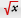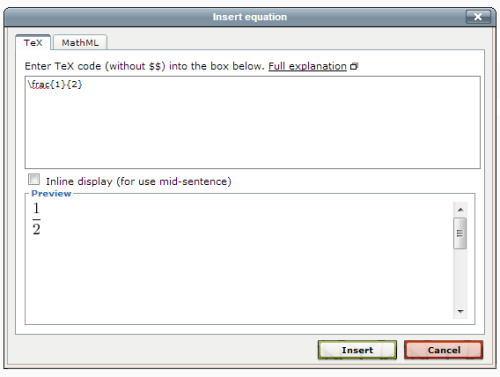# 2.10 Including TeX and MathML

The Maths On-Line Project's preferred way of displaying mathematical equations in the VLE is to use MathML. However writing MathML is not so easy. The alternative is to use TeX.

Within the VLE both TeX and MathML are displayed through a Mathematics service which will also convert between the two, i.e. authors can enter TeX and the mathematics service will create the corresponding MathML. Students may choose to receive their mathematics as images from TeX or as MathML that is interpreted by their browser.

To see a rendition of your maths as you type it use the 'insert equation' tool. Choose the appropriate tab and enter your expression. Here are a couple to try.

This following TeX will render as one half.

\frac{1}{2}2.94 TeX typed into the editor

The equivalent MathML is:

This MathML
<math xmlns="http://www.w3.org/1998/Math/MathML">
<semantics>
<mstyle displaystyle="true">
<mfrac>
<mn>1</mn>
<mn>2</mn>
</mfrac>
</mstyle>
<annotation encoding="application/x-tex">\frac{1}{2}</annotation>
</semantics>
[/itex]
will render as one half.

Note the annotation field. This is used for building the TeX based Alt-text.

Should you wish to include MathML here is a paper on generating MathML with MathType. http://www.dessci.com/ en/ reference/ white_papers/ TUG_paper.pdf [Tip: hold Ctrl and click a link to open it in a new tab. (Hide tip)] .

2.9.3 Audio-visual files